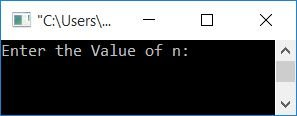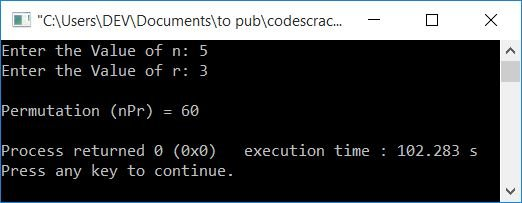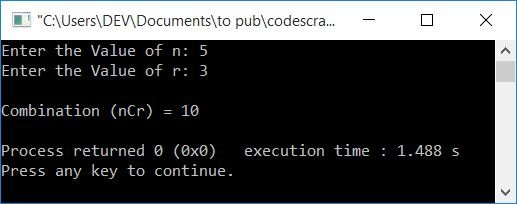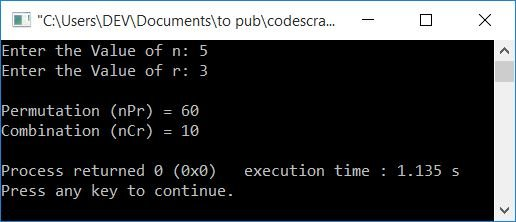# C++ Program to Find nPr and nCr

In this article, you will learn and get code to find and print nPr (permutation) and nCr (combination) in the C++ language. Here is the list of programs available in this article:

Before going through these programs, let's understand the formula used to calculate permutation (nPr) and combination (nCr).

### nPr Formula

The formula to find the value of nPr is:

`nPr = n!/(n-r)!`

Note: The symbol (!) indicates factorial.

Note: The permutation (nPr) value shows the number of ways to arrange r things out of n.

### nCr Formula

The formula to find the value of nCr is:

`nCr = n!/r!(n-r)!`

Note: The combination (nCr) value shows the number of ways to select r things out of n.

## C++: Find nPr (permutation)

To find nPr in C++ programming, you have to ask the user to enter the values of n and r. In both the program for calculating nPr and nCr, the only required code is to find the factorial of a number. The rest of the things are just normal things like initializing, dividing, printing, etc.

The question is, "Write a program in C++ to find the value of nPr." Here is its answer:

```#include<iostream>
using namespace std;
int main()
{
long long fact=1, numerator, denominator;
int perm, n, r, i=1, sub;
cout<<"Enter the Value of n: ";
cin>>n;
cout<<"Enter the Value of r: ";
cin>>r;
while(i<=n)
{
fact = i*fact;
i++;
}
numerator = fact;                    // n!
sub = n-r;
fact = 1;
i = 1;
while(i<=sub)
{
fact = i*fact;
i++;
}
denominator = fact;                // (n-r)!
perm = numerator/denominator;
cout<<"\nPermutation (nPr) = "<<perm;
cout<<endl;
return 0;
}```

This program was built and runs under the Code::Blocks IDE. Here is the sample run:Now type a number, say 5, as the value of n, and then type another number, say 3, as the value of r. Now press the `ENTER` key to find and print the value of nPr as shown in the output given below:There are 60 ways to arrange three things out of five.

The following block of code:

```while(i<=n)
{
fact = i*fact;
i++;
}```

is used to find the factorial of n. So the statement:

`numerator=fact;`

is used to initialized the factorial of n to the numerator. In other words, numerator = n!

In the above program, the factorial of n and n-r gets calculated, and then using the formula of nPr, its value gets calculated and printed on the output. For more information, consult the factorial program.

## C++: Find nCr (combination)

This program asks the user to enter the value of n and r. Based on the values, the program finds and prints the value of nCr, which tells the number of ways to select r things out of n:

```#include<iostream>
using namespace std;
int main()
{
long long fact=1, numerator, denominator;
int comb, n, r, i=1, sub;
cout<<"Enter the Value of n: ";
cin>>n;
cout<<"Enter the Value of r: ";
cin>>r;
while(i<=n)
{
fact = i*fact;
i++;
}
numerator = fact;                    // n!
sub = n-r;
fact = 1;
i = 1;
while(i<=sub)
{
fact = i*fact;
i++;
}
denominator = fact;               // (n-r)!
fact = 1;
i = 1;
while(i<=r)
{
fact = i*fact;
i++;
}
denominator = (fact*denominator);
comb = numerator/denominator;
cout<<"\nCombination (nCr) = "<<comb;
cout<<endl;
return 0;
}```

Here is its sample run with user input, with 5 as the value of n and 3 as the value of r:There are 10 ways to select 3 things out of 5.

## Find nPr and nCr in a Single C++ Program

This program receives the values of n and r. Then it calculates and prints the permutation and combination values for both.

```#include<iostream>
using namespace std;
int main()
{
long long fact=1, numerator, denominator;
int npr, ncr, n, r, i=1, sub;
cout<<"Enter the Value of n: ";
cin>>n;
cout<<"Enter the Value of r: ";
cin>>r;
while(i<=n)
{
fact = i*fact;
i++;
}
numerator=fact;                   // fact = n!
sub = n-r;
fact=1, i=1;
while(i<=sub)
{
fact = i*fact;
i++;
}
denominator = fact;               // fact = (n-r)!
npr = numerator/denominator;
i=1, fact=1;
while(i<=r)
{
fact = i*fact;
i++;
}
ncr = npr/fact;                   // fact = r!
cout<<"\nPermutation (nPr) = "<<npr;
cout<<"\nCombination (nCr) = "<<ncr;
cout<<endl;
return 0;
}```

Here is its sample run with the same user input as provided in the above program's sample run:### Using a for loop, calculate nPr and nCr

This program uses a for loop (instead of while) to do the same job as the previous program.

```#include<iostream>
using namespace std;
int main()
{
long long fact, numerator, denominator;
int npr, ncr, n, r, i, sub;
cout<<"Enter the Value of n: ";
cin>>n;
cout<<"Enter the Value of r: ";
cin>>r;
for(i=1, fact=1; i<=n; i++)
fact = i*fact;
numerator=fact;                   // fact = n!
sub = n-r;
for(i=1, fact=1; i<=sub; i++)
fact = i*fact;
denominator = fact;               // fact = (n-r)!
npr = numerator/denominator;
for(i=1, fact=1; i<=r; i++)
fact = i*fact;
ncr = npr/fact;                   // fact = r!
cout<<"\nPermutation (nPr) = "<<npr;
cout<<"\nCombination (nCr) = "<<ncr;
cout<<endl;
return 0;
}```

This program produces the same output as the previous program.

### Using Function, calculate nPr and nCr.

This is the last program of this article and uses some user-defined functions to do the same task as the previous program.

```#include<iostream>
using namespace std;
long long findFact(int);
int findNPR(int, int);
int findNCR(int, int);
int main()
{
int npr, ncr, n, r;
cout<<"Enter the Value of n: ";
cin>>n;
cout<<"Enter the Value of r: ";
cin>>r;
npr = findNPR(n, r);
ncr = findNCR(n, r);
cout<<"\nPermutation (nPr) = "<<npr;
cout<<"\nCombination (nCr) = "<<ncr;
cout<<endl;
return 0;
}
long long findFact(int num)
{
int i=1, fact=1;
while(i<=num)
{
fact = i*fact;
i++;
}
return fact;
}
int findNPR(int n, int r)
{
long long numerator, denominator;
numerator = findFact(n);
denominator = findFact(n-r);
return (numerator/denominator);
}
int findNCR(int n, int r)
{
int npr, ncr;
npr = findNPR(n, r);
ncr = npr/findFact(r);
return ncr;
}```

#### The same program in different languages

C++ Quiz

« Previous Program Next Program »